Biogeosciences, 15, 4103–4124, 2018
https://doi.org/10.5194/bg-15-4103-2018
Biogeosciences, 15, 4103–4124, 2018
https://doi.org/10.5194/bg-15-4103-2018

Research article 05 Jul 2018

Research article | 05 Jul 2018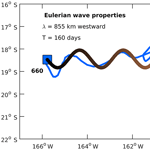# Intermediate water flows in the western South Pacific: as revealed by individual Argo floats trajectories and a model re-analysis

Intermediate water flows in the western South Pacific: as revealed by individual Argo floats trajectories and a model re-analysis
Simon Barbot1, Anne Petrenko1, and Christophe Maes2 Simon Barbot et al.
• 1Aix Marseille Université, CNRS/INSU, IRD, Université du Sud Toulon-Var, Mediterranean Institute of Oceanography (MIO), UM 110, 13288 Marseille, France
• 2Laboratoire d'Océanographie Physique et Spatiale (LOPS), CNRS, Ifremer, IRD, UBO, Brest, France

Correspondence: Simon Barbot (simon.barbot@legos.obs-mip.fr)

Abstract

Thanks to the autonomous Argo floats of the OUTPACE cruise (Oligotrophy to UlTra-oligotrophy PACific Experiment) and of the THOT (TaHitian Ocean Time series) project, some features of intermediate-flow dynamics, at around 1000 m depth, within the central and western South Pacific Ocean (around 19 S, 156 E–150 W) are described. In the Coral Sea, we highlight minima in dissolved oxygen of 140 µmol kg−1 that are associated with the signature of a southward transport of waters between two zonal jets: from the North Vanuatu Jet to the North Caledonia Jet. This transport takes place in the core of a cyclonic eddy or via the path between a cyclonic eddy and an anticyclonic one, highlighting the importance of mesoscale dynamics in upper thermocline and surface layers. Further east, we observe a strong meridional velocity shear with long-term float trajectories going either eastward or westward in the lower thermocline. More interestingly, these trajectories also exhibit some oscillatory features. Those trajectories can be explained by a single Rossby wave of 160-day duration and 855 km wavelength. Considering the thermohaline context, we confirm the meridional shear of zonal velocity and highlight a permanent density front that corresponds to the interface between Antarctic intermediate waters and North Pacific deep waters. Hence both circulation and thermohaline contexts are highly prone to instabilities and wave propagation.

Share
1 Introduction

The intermediate water masses have been known to be of great importance for the thermohaline overturning circulation and for biogeochemical cycles throughout the world's oceans. More specifically, the western tropical South Pacific is of interest to the biogeochemistry community because of its oligotrophic and ultra-oligotrophic zones where diazotrophy has a large influence on phytoplankton growth . To make progress on this subject, the OUTPACE cruise (Oligotrophy to UlTra-oligotrophy PACific Experiment; ), on board the R/V L'Atalante, took place during spring 2015. The surface circulation during the cruise has been studied in order to understand the impact of mesoscale and submesoscale dynamics on an observed bloom and on the horizontal distribution of biogeochemical/biological components , and to place them in the large-scale circulation of the western tropical South Pacific .

Our study will complement these observations of the surface circulation both in space, by focusing on intermediate levels, and in time, by using the time series (more than 2 years) of the autonomous Argo floats deployed during the cruise.

Autonomous Argo floats are profilers parked at 1000 m depth and reaching the surface every 10 days, where they transmit their measurements and location (further information on the cycle of the different Argo floats we used is detailed in the next section). Current speeds at the parking depth of the floats are then calculated, leading to horizontal gridded maps of velocities either global or regional . Other approaches are developed in order to study the intermediate circulation, for example, by taking into account the spreading of different float trajectories from a common position . Moreover, when Argo floats are biogeochemical floats (BGC Argo), on top of allowing the study of intermediate circulation, they provide measurements of biogeochemistry and biology parameters over the first thousand meters of the water column.

In a sense, the studies focusing on the specificity of one particular trajectory (e.g., ) are less frequent now that data from many floats have become available. Our approach focuses on a few float trajectories, using them as witnesses of intermediate waters' specific dynamics.

The cruise started in the Coral Sea, where the main upper currents are characterized by narrow jets named the North Vanuatu Jet (NVJ) and the North Caledonia Jet (NCJ) at the entrance of the Coral Sea. Both of them are westward zonal jets (Fig. 1a; ), but the water masses they carry at the level of the main thermocline are not identical. examine the composition of water masses and report a net difference in dissolved oxygen concentration (DOXY) with waters less oxygenated in the NVJ than in the NCJ. These two jets derive from different branches of the South Equatorial Current (SEC), a broad current with prevailing geostrophic westward currents (Fig. 1a). The NVJ is older, in the sense that it originates from a longer path than the NCJ, regarding its last contact with the surface. Hence, without ventilation, the DOXY of the NVJ intermediate waters is lower than the NCJ DOXY. Using DOXY as a proxy to find the difference between NVJ and NCJ, revealed an intrusion of NVJ waters in the NCJ pathway during the BIFURCATION cruise (Maes2012). The former authors concluded that the NVJ waters were transported southwards by a mesoscale anticyclonic eddy. Here the BGC Argo float deployed at long-duration station A (LDA) of OUTPACE, north of New Caledonia, has followed the path of the NCJ. Changes in the BGC Argo float data allow us to determine whether it has encountered water masses coming from the NVJ.

Further east, other Argo floats, deployed during OUTPACE, are characterized by a main eastward displacement in the opposite direction compared to the westward SEC (Fig. 1a). Observations in other regions of the world have revealed a complex alternation of zonal currents, now known as striations, in surface zonal velocity estimated with altimetric products (e.g., ). These striations have also been found, more recently, at intermediate levels with Argo floats (Fig. 1c; ). More detailed works are underway on such striations in order to observe them more precisely and to understand their physics . During the OUTPACE cruise, highlighted the velocity anomaly of such striations at the surface in one specific area close to the OUTPACE domain (18–22 S, 160 E–150 W). Thanks to the floats, we can extend this observation at intermediate levels and several years after the cruise. In our case, two intermediate water masses are present near the parking depth of the floats, around 1000 m: the Antarctic intermediate waters (AAIWs) to the south and the North Pacific deep water (NPDW) to the north (Fig. 1b).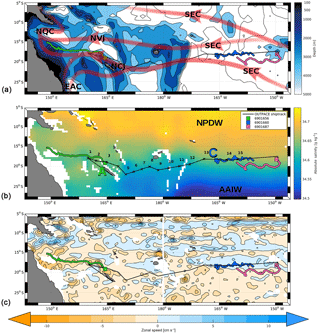Figure 1Western South Pacific Ocean (a) main trajectories of zonal jets superimposed on the bottom topography (in m), (b) absolute salinity (g kg−1) at 1000 m for November climatology from the ISAS13 atlas and (c) zonal mean current (in cm s−1, positive for eastward velocity) deduced from the Argo float displacement at their parking depth (near 1000 m, adapted from ). The colored lines represent the studied floats (WMO number in the inset) with squares for their immersion location. The currents shown in the upper panel are the South Equatorial Current (SEC), the North Vanuatu Jet (NVJ), the North Caledonia Jet (NCJ), the North Queensland Current (NQC) and the East Australia Current (EAC). The water masses shown on the middle panel are the North Pacific deep water (NPDW) and the Antarctic intermediate waters (AAIWs). The black line represents the ship track of the OUTPACE cruise; in the middle panel, the crosses represent the short-time stations (numbers), and the squares A and C represent two of the long-time stations of the cruise where the floats in this study were deployed.

The first part of this study focuses on the mesoscale interactions between NCJ and NVJ within the Coral Sea. Thanks to Argo float WMO6901656, equipped with an optode sensor, we analyze the DOXY variability over the 2015–2016 period using it as a proxy in order to distinguish NCJ waters from NVJ ones. Then, we place the DOXY anomalies in their thermohaline and circulation context. In the second part, we focus on the central Pacific Ocean zone, where we describe specific trajectories of floats deployed during the OUTPACE cruise or in the framework of the THOT project (TaHitian Ocean Time series; ). Once again, we also place the float trajectories in their thermohaline and circulation context. In order to explain their displacement at mid-depths, we choose a wave approach and compare our results, taking into account Doppler shift, to different cases of Rossby, Kelvin and Kelvin–Helmholtz instability waves. Then, we discuss the proposed hypothesis for the two areas and what they imply for the OUTPACE observations. Finally, we conclude and propose some method improvements.

2 Data sets and methodology

## 2.1 Autonomous floats

In the present study we benefit from the deployment during the 2015 spring of several Argo floats (https://doi.org/10.17882/42182, Argo, 2000) under the auspices of the OUTPACE cruise and of the THOT project . We analyze the first 2 years of data. All OUTPACE and THOT floats are related to the Argo international program and have the same type of sampling cycle. This cycle starts with the descent of the float to a depth of around 1000 m, called the parking depth, where the floats drift for a programmed time. This time can be remotely modified in the latest generation of floats, such as those used in this study. Here we use the measurements made while the floats are rising to the surface. At the surface, these data are transmitted via satellite. Even if this cycle is the same for all floats, the time spent at the parking depth (in our cases, 5 or 10 days) and hence the corresponding sampling frequency (Δt) are not the same for all of them. Two types of float are available: ARVOR (Argo Core) floats and PROVBIO floats (Bio-Argo). Because they were all deployed during the same months, they all have a close WMO code: #6901XXX. Hereafter, we only use the last three digits of the float number to refer to them; e.g., float 656 refers to #6901656. In this study, we use three PROVBIO floats using Iridium (656, 660 and 687) and two ARVOR floats using ARGOS (671 and 679). Details and data are accessible via the Coriolis operational center (http://www.coriolis.eu.org, last access: 7 March 2017). In order to get the average velocity of the floats during their cycles, we follow the method of , taking different time slots over the float cycle in order to calculate, for the nth cycle, the surface velocity Vn(0) and the velocity at 1000 m Vn(1000):

$\begin{array}{}\text{(1)}& {V}^{n}\left(\mathrm{0}\right)=\frac{{L}_{\mathrm{last}}^{n}-{L}_{\mathrm{first}}^{n}}{{t}_{\mathrm{last}}^{n}-{t}_{\mathrm{first}}^{n}},\phantom{\rule{1em}{0ex}}{V}^{n}\left(\mathrm{1000}\right)=\frac{{L}_{\mathrm{first}}^{n}-{L}_{\mathrm{last}}^{n-\mathrm{1}}}{{t}_{\mathrm{first}}^{n}-{t}_{\mathrm{last}}^{n-\mathrm{1}}},\end{array}$

where Lfirst and Llast are the first and the last transmitted location in the same cycle and t is the corresponding time.

Table 1Statistics for the different floats between surface and deep trajectories; the results are presented as “mean ± SD”.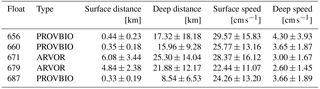Table 1 shows the mean properties of displacements for each studied float. First, it highlights the differences in surface distances between PROVBIO and ARVOR floats. At the surface, ARVOR floats drift over a distance about 10 times greater than PROVBIO floats. This is due to the longer time they spend at the surface (6 h for ARVOR floats and 24 min for PROVBIO floats). The ratio between deep and surface distances is a factor of 30 for PROVBIO floats and still 4 for ARVOR ones. Float 656 exhibits an anomalously high standard deviation for its deep distance and deep speed. These high values, on the same order as the mean ones, are due to the period during which the float was grounded on the sea floor on the Queensland Plateau. Otherwise we have concluded that surface displacement can be neglected compared to the deep displacement without doubt for PROVBIO floats and with caution for ARVOR floats. Hence, when necessary in the wave section, we will only use the former ones and consider that the trajectory dynamics are mainly due to deep circulation processes. The discussion of such consideration is thoroughly made by .

In the case of the 656 float, we also consider the DOXY measurements. These data were calibrated (Alain Fumenia, personal communication, March 2017) based on the CTD profiles made just before the float was deployed during the OUTPACE cruise. The calibration for the 656 float is

$\begin{array}{}\text{(2)}& {\text{DOXY}}_{\mathrm{clb}}=\mathrm{0.97125}\phantom{\rule{0.125em}{0ex}}{\text{DOXY}}_{\mathrm{raw}}+\mathrm{14.3755},\end{array}$

where DOXYraw is the dissolved oxygen concentration data from the float and DOXYclb is the calibrated dissolved oxygen concentration data, both expressed in micromoles per kilogram (µmol kg−1).

## 2.2 Wave characteristics from float trajectories

Here the objective is to find the characteristics of a single wave that could explain the float trajectories which represent both retrograde and prograde circulation (retrograde when Lagrangian motion is in the opposite direction compared to the wave propagation; prograde when Lagrangian motion and wave propagation are in the same direction; as defined in ).

Thus, we describe the float trajectories as Lagrangian description of waves, using the period T and the wavelength λ as well as the frequency ω ($\mathit{\omega }=\mathrm{2}\mathit{\pi }/T$) and the wave number k ($k=\mathrm{2}\mathit{\pi }/\mathit{\lambda }$). In our case, the dynamics of the floats do not allow us to extract information concerning the vertical component because data on float locations are only measured at the surface. The displacements of the floats are mostly directed by the currents, and only secondarily by waves. Since the prevailing currents are mainly zonal, we consider hereafter, to simplify, that the wave numbers derived from the observations correspond to the longitudinal component (k=klon). To clarify the methodology, we choose to name the measured wavy trajectory of a float “float wave” and the process that could lead to such trajectory “theoretical wave”.

We tried to describe the float waves with a Fourier transform or a wavelet analysis on the float time series, but the description of the different frequencies contained in them was incomplete. This is due to the shortness of the time series (2 years) with regard to the sampling period. If the floats are still functional in a few years, these methods should be reconsidered.

So instead, we developed the method presented here, with Lagrangian and Eulerian wave descriptions and the estimations of wave numbers. In order to maximize the information derived from the portions of the trajectories where the floats oscillate, we choose not to describe the complete float wave characteristics (T, λ) but rather their halves (T∕2, λ∕2). In practice, this means we measure time and distance from crest to trough (and so on) rather than from crest to crest, for each studied float (floats 660, 671, 679 and 687; Fig. 2). Hence, it allows for more estimates when incomplete cycles are present. The measurements of λ∕2 are made from the position maps and of T∕2 from the float time series of latitude.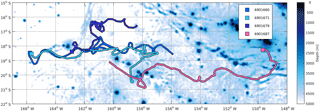Figure 2Trajectories of the studied floats superimposed on bottom topography (in m). The squares represent the initial location of the floats.

Because the floats are, by definition, Lagrangian devices, we need to be careful before comparing the float waves to the classical Eulerian oceanic waves. We first use a simple case to get a basic relation between Eulerian wave parameters and Lagrangian ones. Because of the zonal tendency of the studied float trajectories (Fig. 2), we express a simple case of the current perturbations due to a homogeneous, monochromatic wave (hereafter plane wave) propagation with the following system of equations:

$\begin{array}{}\text{(3)}& \left\{\begin{array}{l}u={u}_{\mathrm{0}}\\ v={v}_{\mathrm{0}}\phantom{\rule{0.125em}{0ex}}\mathrm{sin}\left({\mathit{\omega }}_{\mathrm{Te}}t-{k}_{\mathrm{Te}}x+{\mathit{\phi }}_{\mathrm{Te}}\right)\end{array}\right\,\end{array}$

where u0 and v0 are zonal and meridional velocities considered as constants, ωTe is the frequency, kTe is the wave number and φTe is the phase shift term of the Eulerian theoretical wave. Note that v0 is the amplitude ATe of the Eulerian theoretical wave. By convention, the subscript “T” is used for theoretical, “M” for measured, “e” for the Eulerian description of the wave and “” for the Lagrangian one (Table 2).

Table 2Summary of the different notations of the wave properties: frequency ω, wave number k and amplitude A.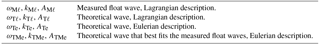After performing some tests (Appendix A), we choose to set the zonal velocity of the floats as the global mean velocity of the float trajectory:

$\begin{array}{}\text{(4)}& {u}_{\mathrm{0}}=\left\{\begin{array}{ll}\frac{{L}^{\mathrm{end}}-{L}^{\mathrm{1}}}{{t}^{\mathrm{end}}-{t}^{\mathrm{1}}}=\mathrm{1.65}\phantom{\rule{0.125em}{0ex}}\mathrm{cm}\phantom{\rule{0.125em}{0ex}}{\mathrm{s}}^{-\mathrm{1}}& \text{for float 660}\\ & \\ \frac{{L}^{\mathrm{end}}-{L}^{\mathrm{58}}}{{t}^{\mathrm{end}}-{t}^{\mathrm{58}}}=-\mathrm{1.93}\phantom{\rule{0.125em}{0ex}}\mathrm{cm}\phantom{\rule{0.125em}{0ex}}{\mathrm{s}}^{-\mathrm{1}}& \text{for float 687}\end{array}\right\,\end{array}$

where Lend is the location of the float in the last cycle. For float 687, the 58th cycle corresponds to the start of the float's westward propagation. Thus, we only consider the 687 float trajectory from the 58th cycle to the end location.

In such a configuration, the Lagrangian observation of the float can be represented by a Doppler effect on the perception of the theoretical wave. So we can express the Lagrangian properties of the theoretical wave (ωTℓ, kTℓ and ATℓ) as a function of ωTe, kTe, ATe and u0:

$\begin{array}{}\text{(5)}& \left\{\begin{array}{l}{\mathit{\omega }}_{\text{T}\mathrm{\ell }}={\mathit{\omega }}_{\mathrm{Te}}-{k}_{\mathrm{Te}}{u}_{\mathrm{0}}\\ {k}_{\text{T}\mathrm{\ell }}={k}_{\mathrm{Te}}-\frac{{\mathit{\omega }}_{\mathrm{Te}}}{{u}_{\mathrm{0}}}\\ {A}_{\text{T}\mathrm{\ell }}=-\frac{{A}_{\mathrm{Te}}}{{\mathit{\omega }}_{\mathrm{Te}}-{k}_{\mathrm{Te}}{u}_{\mathrm{0}}}\end{array}\right\.\end{array}$

But these equations derive from one common expression; hence this system cannot be solved like one with three equations and three unknowns. Solving Eq. (5) is trivial only when setting either ωTe or kTe. As we want to estimate both of them, we need another method to find the Eulerian properties of the theoretical wave that best fits the float trajectories. Hereafter, we use those equations in order to build an index I for the differences between the Lagrangian properties of the float waves (ωMℓ and kMℓ) and the Lagrangian properties of the potential theoretical waves (ωTℓ and kTℓ). I is defined by the following expression:

$\begin{array}{}\text{(6)}& {I}_{a}=\mathit{\left\{}\left|{\mathit{\omega }}_{\text{M}\mathrm{\ell }}-{\mathit{\omega }}_{\text{T}\mathrm{\ell }}\right|+\left|{u}_{\mathrm{0}}\left({k}_{\text{M}\mathrm{\ell }}-{k}_{\text{T}\mathrm{\ell }}\right)\right|{\mathit{\right\}}}_{a},\end{array}$

where a is the float considered.

We made the calculations for a range of Eulerian frequencies and wave numbers (ωTe and kTe), thanks to Eq. (5), in order to get the diagrams of this index for float 660 (I660, Fig. 3a) and for float 687 (I687, Fig. 3b). For each index, the zone gathering all the index minima has a linear shape: positive for float 660 and negative for float 687. Hence, since these linear slopes are of opposite signs, minimizing the sum of the two indices leads to finding where the two lines of minima cross. The crossing approach determines the properties of the theoretical wave that could best fit both the float trajectories. This method could be used with several other floats and be expressed by the following formulation:

$\begin{array}{}\text{(7)}& I=\sum _{na=\mathrm{1}}^{N}{I}_{na},\end{array}$

where N is the total number of floats considered, in our study N=2. Using this numerical approach, we can find all the couples (ωTe, kTe) that minimize I and, hence, that could fit the float trajectories.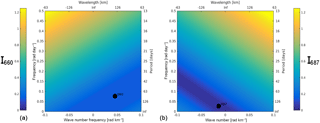Figure 3Difference between observed Lagrangian float wave parameters and Lagrangian theoretical wave ones (index I) for (a) float 660 and (b) float 687. Negative wavelengths describe westward waves. Black dots indicate the observed Lagrangian parameters.

In order to compare the couples that minimize I and find the one that best fits the 660 and 687 float trajectories, we run a simulation of the idealized trajectory for each couple. The simulations use Eq. (3), integrating them to express the longitude x(t) and the latitude y(t) of Lagrangian particles as a function of time:

$\begin{array}{}\text{(8)}& \left\{\begin{array}{ll}x\left(t\right)& ={x}_{\mathrm{0}}+{u}_{\mathrm{0}}t\\ y\left(t\right)& ={y}_{\mathrm{0}}+\frac{{v}_{\mathrm{0}}}{{\mathit{\omega }}_{\mathrm{Te}}-{k}_{\mathrm{Te}}{u}_{\mathrm{0}}}\left[\mathrm{cos}\left(\left(\frac{{\mathit{\omega }}_{\mathrm{Te}}}{{u}_{\mathrm{0}}}-{k}_{\mathrm{Te}}\right)x\left(t\right)\\ & -\frac{{w}_{\mathrm{Te}}}{{u}_{\mathrm{0}}}{x}_{\mathrm{0}}+{\mathit{\phi }}_{\mathrm{Te}}\right)-\mathrm{cos}\left(-{k}_{\mathrm{Te}}{x}_{\mathrm{0}}+{\mathit{\phi }}_{\mathrm{Te}}\right)\right]\end{array}\right\,\end{array}$

where x0 and y0 define the initial position of the float. The simulations run with a time step of 1 day for 642 days (${\left\{{t}^{\mathrm{end}}-{t}^{\mathrm{1}}\right\}}_{\mathrm{660}}={\left\{{t}^{\mathrm{end}}-{t}^{\mathrm{58}}\right\}}_{\mathrm{687}}=\mathrm{642}$ days in Eq. 4). In order to compare the simulations to the float trajectories, we interpolate the latitudes of the float, yint, at the longitudes of the corresponding simulations. Then we calculate the sum of the differences of latitude using an index J, defined as

$\begin{array}{}\text{(9)}& {J}_{a}=\mathit{\left\{}\sum _{j=\mathrm{1}}^{m}|\phantom{\rule{0.25em}{0ex}}{y}_{\mathrm{int}}\left(j\right)-{y}_{\mathrm{simu}}\left(j\right)\phantom{\rule{0.25em}{0ex}}|{\mathit{\right\}}}_{a},\end{array}$

where a is the considered float; ysimu are the latitudes of the simulation; and m is the total number of days, here m=642. Because the value of φTe can influence the value of J, we carried out several simulations for each couple (ωTe, kTe), varying φTe from π to π with a step of $\frac{\mathrm{1}}{\mathrm{10}}\mathit{\pi }$. As for index I, we can sum J660 and J687 to get the couple that minimizes the trajectory differences of both floats 660 and 687. Because the initial time of the two floats is the same, φTe must be the same for the two floats. Then we sum J660 and J687 for each φTe. This method could also be used with several other floats and be expressed by the following equation:

$\begin{array}{}\text{(10)}& J=\sum _{na=\mathrm{1}}^{N}\frac{{J}_{na}}{{\mathit{\sigma }}_{na}},\end{array}$

with σ being the standard deviation of the latitude of the float.

These two steps give us the couple (ωTMe, kTMe) of the theoretical wave that best fits the trajectories of floats 660 and 687. This couple can now be compared to classical Eulerian oceanic waves such as Kelvin, Rossby and Kelvin–Helmholtz instability waves.

According to , for each float, we can define

$\begin{array}{}\text{(11)}& \mathit{\epsilon }=\frac{{u}_{\mathrm{0}}}{c}\phantom{\rule{0.33em}{0ex}},\end{array}$

where c is the phase speed of the wave ($c=\frac{\mathit{\omega }}{k}$). The sign of ε indicates the type of motion of the float (prograde or retrograde), and its amplitude compares the particle velocity to the wave speed.

## 2.3 Ancillary data

In order to evaluate the possible link between oxygen anomalies and surface currents in the Coral Sea, we use the AVISO altimetry products (Archiving, Validation and Interpretation of Satellite Oceanographic data; http://www.aviso.altimetry.fr, last access: 20 January 2017). We select ocean-level elevation anomaly products, reworked from all satellites, to obtain geostrophic velocity anomaly fields (ug and vg) with a 1∕4 resolution. From those data we calculate the geostrophic velocity amplitude. We also use HYCOM (HYbrid Coordinate Ocean Model) re-analysis GLBu0.08 of the experiment 91.1 from March 2015 to March 2016 (https://www.hycom.org, last access: 4 June 2018). This system is a hybrid isopycnal–sigma–pressure (generalized) coordinate ocean model with 1∕12 horizontal resolution and 40 vertical levels assimilating in situ data.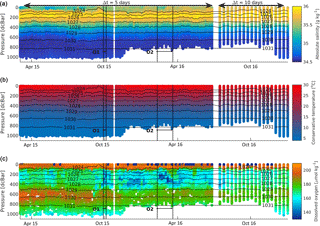Figure 4Profiles of float 656 over depth and time for (a) absolute salinity, (b) conservative temperature and (c) dissolved oxygen concentration. Every colored point corresponds to a measurement. The horizontal black lines indicate the isopycnals from 1024 to 1031 kg m−3. The vertical black lines indicate the limits of the low-oxygen events O1 and O2. Over the top of (a), Δt indicates the two different sampling frequencies of the float.

In order to place our analyses within the global thermohaline context, we used the ISAS13 atlas (In Situ Analysis System; ), which provides a climatology of thermohaline properties. This atlas collects and processes all the profiles provided by the Argo floats from 2004 to 2012 in order to calculate a monthly global climatology over the entire depth of the oceans. More details are provided by . We apply the same methods to calculate the density as those used for the HYCOM re-analysis.

In the following, whatever is the source for the temperature and salinity fields, the density of the water masses is computed with the Matlab toolbox Gibbs Seawater based on the TEOS-10 convention (http://www.teos-10.org/software.htm, last access: 10 April 2017).

3 Results

## 3.1 Coral Sea

The trajectory of float 656 can be clearly associated with the flow along the New Caledonian coasts at the entrance of the Coral Sea (; Fig. 1a). The float then travels westward until the Queensland Plateau, where it often reaches the bottom before stabilizing near its parking depth. Hence, from November 2015 to October 2016, it either moves very slowly or stays stuck in the same region. Afterwards the float moves northwestward, circumventing the plateau at the end of the period analyzed here. This trajectory corresponds very strongly with the NCJ pathway.

### 3.1.1 The DOXY variability of the jets

The correspondence between Argo profiles and the HYCOM re-analysis for conservative temperature, absolute salinity and density can be found in Appendix C. As expected from a re-analysis that assimilates different measurements including Argo float data, the salinity and temperature are in good agreement with the float measurements.

In addition to the standard parameters of conservative temperature and absolute salinity, float 656 provides DOXY profiles. We plot the temporal evolutions of these properties, corresponding to a mainly east–west transect (Fig. 4). Whereas temperature and salinity vary quite homogeneously below the upper layers, we can observe a strong oxygen stratification of the water column: higher concentrations at the surface (around 200 µmol kg−1) and between 500 and 900 m (around 190 µmol kg−1), and lower concentrations between 100 and 500 m and below 900 m (around 170 µmol kg−1). Stratification is only partially found in the salinity profiles: lower concentrations at the surface (around 35.5 g kg−1) and below 400 m (less than 35.25 g kg−1) and higher concentrations between 100 and 400 m (around 36 g kg−1).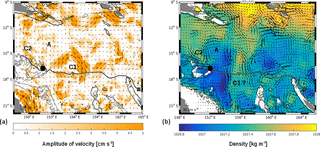Figure 5(a) Amplitude of surface geostrophic velocity on 29 February 2016 during O2, calculated from AVISO product; (b) density and velocity field at 300 m depth on 29 February 2016 during O2, calculated from the HYCOM re-analysis. The gray line corresponds to the full trajectory of float 656, and the black point to the location of the float at the stated date. The black lines correspond to the bottom topography at 300 and 1000 m depth.

In the intermediate layers, between 100 and 500 m, we notice a strong variability in the oxygen, and especially strong pulses of low values. We isolated two strong low-oxygen events (O1 and O2) with values below 140 µmol kg−1. O1 is an event of about 1 month covering October 2015 with measurements between 150 and 140 µmol kg−1. O2 is spread over 2 months from February to March 2016 with measurements lower than 130 µmol kg−1. The DOXY signatures of O1 and O2 do not correspond to the classical characteristics of the NCJ , whereas the float is exactly on its pathway. We therefore seek to know its origin. Based on the work of and , we hypothesize that this signature originates from the NVJ waters whose theoretical and observed pathway is located 4 further north (Fig. 5b). The figure of Appendix B shows the depth variability of the DOXY minimum of each profile during O2 and helps to elucidate the link between the low-oxygen events and the properties of NVJ waters.

### 3.1.2 Thermohaline and circulation context

We focus on O2, which is longer and stronger than O1. The geostrophic velocity fields from AVISO (Fig. 5a) allow us to visualize the position of the float during O2 in relation to the surface circulation. We notice that, during the whole duration of O2, the float is located on the Queensland Plateau, where we can note that the deepest measurements are shallower than the parking depth (also visible in Fig. 4a, b, c). This leads to short displacements over the whole event, making the interpretation of the results easier. We can identify a very large cyclonic structure to the east of the plateau centered at 156 E, which we name C1, and a much weaker cyclonic structure, C2, located to the northwest of the plateau. Between them, we observe an anticyclonic structure, A, centered at 153 E and stretching from 13 to 17 S. The western branch of A (152 E) forms a meridional path with southward velocities.

Using the HYCOM re-analysis, we can compare the AVISO observations to the modeled velocity field at different depths. At 300 m, the depth of the DOXY minimum, we can clearly identify structures C2 and A, but C1 is harder to locate (Fig. 5b). C1 may be located 1 farther south. A is much more circular in the HYCOM re-analysis than in the AVISO surface data. These data also allow us to consider the densities of these water masses and thus enable us to track the potential NVJ waters down to the NCJ pathway. Figure 5b clearly shows that the NVJ waters can be associated with C2 for instance.

Otherwise, when studying the AVISO product and the HYCOM re-analysis for O1, we do not identify an eddy structure or a circulation shape that could explain that DOXY anomaly. Nevertheless, due to the large-scale features of water masses and the low values of DOXY, shown in Fig. 4c, we could hypothesize that such low-oxygen events are related to the intrusion of waters transported by mesoscale eddies or by NVJ meanders. Because we can observe such circulations with AVISO products, they definitely affect the surface layers and then impact the studied diazotrophic zones of the OUTPACE cruise. In Sect. 4.1, we will further discuss such aspects.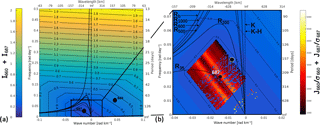Figure 6Difference between observed Lagrangian float wave characteristics and Lagrangian theoretical wave ones (index I, left color bar) for (a) the sum of floats 660 and 687 and (b) the associated minimum calculations (normalized index J, right color bar) with regard to dispersion equations of Rossby wave (solid line), Kelvin wave (dotted line) and Kelvin–Helmholtz instability wave (break line). Subscript “b” denotes a barotropic case; subscripts with a number denote a baroclinic case with stratification at the depth of this number. A negative wavelength describes a westward wave. Black dots indicate the observed Lagrangian parameters, of which in (b) only 687 are inside the range; the red dots show the minima of the index and hence the best parameters that can explain both trajectories; the black dot encircled in white is the couple (ωTMe, kTMe) that best fits the 660 and 687 float trajectories.

It should be noticed that it still remains difficult to interpret observations of different nature, i.e., from an Eulerian versus Lagrangian point of view, and that further work is required to place the float observations in their complete dynamical context.

## 3.2 Central Pacific Ocean

### 3.2.1 Float waves description and Doppler shift correction

In this part we focus on the trajectories of three OUTPACE floats (660, 671 and 679) moving eastward and one THOT float (687) moving westward. These floats were all deployed in spring 2015 and now show, after 2 years of drifting, zonal wavy trajectories (Fig. 2). We consider this group of OUTPACE floats distinctly from the THOT float because of their different directions. Then, we compare their respective float wave characteristics.

Table 3Period T and wavelength λ of the float waves. The mean, standard deviation and extrema values are calculated for the eastward floats: 660, 671 and 679 (top of the table). Then details are given for each float: dates, geographical area, half periods, half wavelengths and corresponding mean and standard deviation values for T and λ.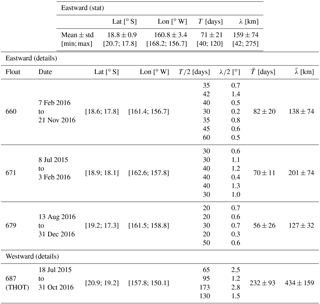The wave characteristics of the two groups (Table 3) are clearly different: 71-day period and 159 km wavelength for the eastward group and 232-day period and 434 km wavelength for the westward float. Based on the couple (ωMℓ, kMℓ) and following the method described in Sect. 2.2, we first estimate the Eulerian characteristics of the theoretical waves (ωTe, kTe and ultimately ωTMe, kTMe) that best fit the floats.

To simplify the presentation, we only use the 660 and 687 float wave properties. We choose these two floats because they are the ones with the most regular trajectories (neither going northward like float 679 nor southward like float 671). As explained in Sect. 2.2, since the two regions of minima cross, the two observed float waves can be the signature of a single theoretical wave. Considering this hypothesis, we find that this wave is defined in the ranges from 0 to 0.075 rad day−1 for the frequency and from 0.04 to 0.02 rad km−1 for the wave number (Fig. 6a). In order to get a better resolution and minimize the calculation time, we zoom in on those ranges of frequencies and wave numbers before doing the minimum calculation. We set a resolution of $\mathrm{5}×{\mathrm{10}}^{-\mathrm{5}}$rad day−1 and $\mathrm{5}×{\mathrm{10}}^{-\mathrm{5}}$rad km−1 and find 8083 minima, mostly in the westward region (Fig. 6b).

In order to compare the couples (ωTe, kTe) to classical oceanic waves, we calculate the dispersion equation of Kelvin and Rossby in a vertical barotropic case, Kelvin–Helmholtz instability wave in a two-layer case and Rossby waves in several baroclinic cases (the different cases are explained in Appendix D). We observe that the characteristics of the Kelvin and Kelvin–Helmholtz instability waves are not in the same ranges as the ones of the couples we want to identify. Rossby waves (R) are the ones that best fit them. The barotropic case Rb is out of range, but most solutions are located around the curves of the baroclinic cases with a thermocline at 35 m (R35) and 200 m (R200).

Using the J index, we are able to select the couple that best fits both the 660 and the 687 float trajectories. The results give us a wave of 160-day duration and 855 km wavelength; the validity of this result will be discussed in the next section. Figure 7 illustrates the agreement between the observed and the reconstructed trajectories obtained from it. Obviously, some other processes with small-scale signatures also influence the observed float trajectories. Nevertheless, a single wave, added to the floats' respective zonal background currents, can mainly explain the two float trajectories that would otherwise be classified, at first sight, as behaving differently. Such observations of a potential plane wave have been rarely highlighted so far, and even less at such a depth.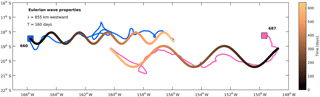Figure 7Best analytical solution of Lagrangian trajectories for both floats 660 and 687. The blue line refers to float 660, and the pink one to float 687; the squares refer to their initial location. The black to brown lines refer to the best theoretical solution with a time step of 1 day.

With the best-fitting wave characteristics (ωTMe, kTMe), we can calculate the phase speed of the wave and the diagnostic ε for each float. Hence $c=-\mathrm{6.2}$cm s−1, ${\mathit{\epsilon }}_{\mathrm{660}}=-\mathrm{0.27}$ and ε687=0.31. As expected, ε660<0 means the float 660 is in a retrograde context, and ε687>0 means the float 687 is in a retrograde one. The value of ε is still very weak ($-\mathrm{1}<\mathit{\epsilon }<\mathrm{1}$) in both context. This attests that we are in a case of non-trapping of the float by the wave (Flierl1981).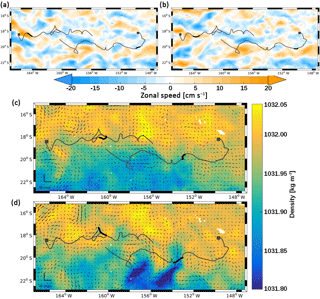Figure 8(a, b) Zonal velocity (positive for eastward velocity) at 1000 m depth on (a) 1 July 2015 and (b) 16 July 2015 from the HYCOM re-analysis; (c, d) density and velocity field at 1000 m depth on (c) 29 September 2015 and (d) 13 November 2015 calculated from the HYCOM re-analysis. The gray lines correspond to the full trajectory of the floats and the black portions to the location of each float at the stated date and on the following 15 days.

### 3.2.2 Thermohaline and circulation context

Using the HYCOM re-analysis, we are able to place the trajectory of the floats into the circulation at 1000 m depth. Figure 8a and b highlight the current striations as mentioned in the Introduction. We observe that the modeled striations have, on average, widths of 1 to 1.5 of latitude, which are smaller than those observed by (Fig. 1c). The mean shear of zonal velocity observed by floats 660 and 687 is equal to 4.2 cm s−1 (zonal velocities used in Appendix A for case (b) in Table 1A of Appendix A).

We also use the HYCOM re-analysis fields of temperature and salinity in order to calculate the density context of the studied area (Fig. 8c). The two floats 660 and 687 are both at the interface of two different water masses: the northern one with a density around 1032 kg m−3 and the southern one with a density around 1031.85 kg m−3. This corresponds to a density difference of 0.15 kg m−3 in a 2 latitudinal range with the isopycnals being deeper southward. The front location, shape and intensity are variable with some extreme events of displacement or intensity, such as on 13 November 2015 (Fig. 8d). But the front usually stays a zonal boundary located around 20 S, such as on 29 September 2015 (Fig. 8c).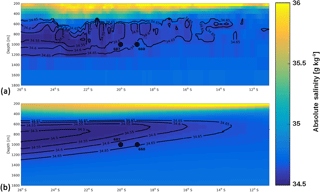Figure 9Absolute salinity meridional section at 157 W calculated from (a) the HYCOM re-analysis on 13 November 2015 and (b) the ISAS13 atlas for November seasonal climatology. Black dots indicate the mean location of the floats.

Meridional cross sections of the HYCOM re-analysis (Fig. 9a) show a salinity gradient in the intermediate waters, between 500 and 1200 m. We can note the meridional gradient of salinity at the parking depth of the float, around 1000 m: saltier (35 g kg−1) north of 19 and less salty (34.5 g kg−1) south of 19. These values are also observed in the ISAS13 climatology (Fig. 9b). To quantify the meridional slope of this front, we analyze the depth of the 1031.95 kg m−3 isopycnal (average between the two sides of the front) from 15 to 25 S and from 147 to 167 W. Then we perform a linear regression on the isopycnal depths in their steepest part between 17 and 22 S. We can obtain, for example, low values of 3.8 m per degree of latitude on 13 November 2015 (Fig. 8d), average values of 4.2 m per degree of latitude on 29 September 2015 (Fig. 8c) and what we call extreme values such as 26.9 m per degree of latitude on 13 November 2015. To verify if the meridional slope varies over the year, we have done the calculations for the complete ISAS13 climatology (Table 4). These slope values range from a minimum of 3.9 m per degree of latitude in June to a maximum of 4.5 m per degree of latitude in February. They do not exhibit any apparent annual cycle, and the density front appears to be a permanent feature.

Table 4Meridional slope of the 1031.95 kg m−3 isopycnal in the monthly climatology of the ISAS13 atlas.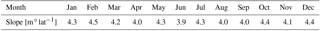Otherwise, we note that, from May to July 2015, a cyclonic eddy (centered at about 18.5 S, 165 W in Fig. 8a, b) crossed the trajectory of float 660. Hence, it is likely that this eddy influenced the beginning of the float 660 trajectory and produced a certain variability in its oscillations. Concerning the trajectory of float 687, we do not observe any stable eddy structure around it during the whole period. That explains why we did not choose to interpret the wavy float trajectories with the unique hypothesis of eddy impacts.

4 Discussion

## 4.1 Coral Sea

When analyzing the DOXY measurements of float 656, we were able to describe two distinct events with well-marked oxygen minima (O1 and O2, Fig. 4c) between 150 and 400 m depth, i.e., near the upper part of the main thermocline. Inspired by the previous study of , we associate these oxygen minima with the NVJ waters, much less oxygenated than those of the NCJ. We propose that the NVJ waters were indeed transported by mesoscale eddies. To support this hypothesis, we compare the geostrophic currents at the surface with AVISO data and also use the HYCOM re-analysis at 300 m. In the case of O2 we have been able to identify a cyclonic structure (C2, Fig. 5) and an anticyclonic structure (A1) in the two sets of data. These vortex structures are involved in the transport of NVJ waters southward to the NCJ pathway. Maps of density resulting from the HYCOM re-analysis inform us about the nature of the cores of these vortex structures. Structure A carries NCJ waters, while C2 transports NVJ waters. This clear difference forms a density gradient of 0.6 kg m−3 over 3 of longitude approximately. From this, we can hypothesize that the signature of the oxygen minimum is due to C2 carrying NVJ waters in its core. Looking at the evolution of the velocity field, we note that the common branch of C2 and A forms a local southward current exactly toward the position of the float 656. This current could also play a role in the transport of NVJ waters. Hence, we propose a second hypothesis of an edge transport for the NVJ waters going south. These waters could be first carried by the northern edge of A and then transported southward thanks to the current located between A to the east and C2 to the west. The first hypothesis fits the results of , with the difference that the structure transporting NVJ waters is cyclonic and not anticyclonic. Hence the two possibilities that we propose broaden the understanding of the connection processes between the NVJ and the NCJ and suggest that there be an explicit consideration of mesoscale eddy variability in future modeling approaches.

Thanks to the analysis of the OUTPACE observations, hypothesize that the location of nitrogen sources and sinks could be decoupled. Thus, the authors propose that the transport of nitrogen-rich thermocline waters from N2 fixation could join the subtropical gyre through the East Australia Current (EAC, Fig. 1a). also observe a westward increase of turbulence during OUTPACE. This leads to a strong turbulent regime in the Melanesian Archipelago, located at the entrance of the Coral Sea, highly visible in nitrogen measurements at the long-term stations. Extending these conclusions to the Coral Sea creates a context favorable to the exchange between NVJ and NCJ. Specific exchanges from NVJ to NCJ would, then, strengthen the recirculation in the subtropical gyre of nitrogen-rich waters. Thus understanding their dynamics could help us to better understand their impact on the propagation of biogeochemical components.

## 4.2 Central Pacific Ocean

The trajectories of OUTPACE and THOT Argo floats give us two groups of float waves with different characteristics. Whereas the long-term mean displacement of the floats could be explained by the presence of alternating striations as deduced from the displacement of the Argo floats at their parking depth, we focused on their quite-surprising oscillation characteristics. In this study, we show that their oscillating trajectories can be caused by a single theoretical wave of 160-day duration and 855 km wavelength superimposed on the zonal background current. In Fig. 7, the fit over the trajectory of float 687 is very convincing. It is less so for float 660. We can explain this by recalling that the beginning of the 660 trajectory is, partially or entirely, influenced by an eddy passing through (Fig. 8a, b). Another explanation could be that, in Eqs. (3) and (4), we consider the zonal velocity u0 as constant during the entire simulations. But a variable zonal velocity is likely to strongly impact the float trajectory. We detail this sentence with two examples that can be observed in the 660 and 687 trajectories. The first one takes place in the 660 trajectory between 159 and 158 W (Fig. 7). It happens when the zonal velocity decreases while keeping the same direction eastward (Fig. 10a). This leads to a smaller local wavelength. The second one takes place in the 687 trajectory around 158 W. It happens when the zonal velocity changes direction, from westward to eastward (Fig. 10b). This leads to a loop in the Lagrangian trajectory. The impact of a variable zonal velocity does not refute our hypothesis of a single wave influencing the two floats 660 and 687, but it questions the precision of the values found (ωTMe, kTMe). The same comment also applies to the description of the couples (ωMℓ, kMℓ)660 and (ωMℓ, kMℓ)687 that could not be performed using a Fourier transformation or a wavelet analysis due to the short duration of the float data time series compared to the sampling frequency. Using a plane wave description is a source of inaccuracy because such waves are rarely observed in open-ocean studies. Some propositions for further analyses are detailed in the next section.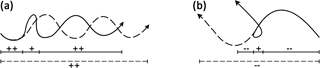Figure 10Sketch explaining the influence over a Lagrangian trajectory of (a) a zonal velocity decrease like in the 660 trajectory and (b) a zonal velocity inversion like in the 687 trajectory. The horizontal dashed lines refer to the trajectories influenced by a wave with a constant zonal velocity. The horizontal solid lines are segmented with the periods associated with the sign above them. The signs indicate the strength and the direction of the zonal velocity: ++ for strong eastward, + for weak eastward and $--$ for strong westward.

Based on the HYCOM re-analysis, we confirm that both the 660 and the 687 floats move in shear environments. This shear context is supported by a density front which is permanent all year round. This front could be associated with the base of the northern edge of the Antarctic intermediate waters that reach this latitude near our study zone . The best-fitting wave that we found is close to a Rossby wave in a baroclinic ocean case with a thermocline at 200 m. This case is close to the real context of the studied zone where the upper thermocline waters are located around 200 m (GLODAPv2 database; ). Moreover, this zone is precisely located where Rossby waves are frequently found, and their signatures can be observed through sea level anomalies . Those arguments strengthen the hypothesis of a Rossby wave close to 160 days in duration and with a 855 km wavelength. These observations lead to a large discussion of the possible interactions between waves and intermediate flows.

Such processes could have a significant impact on the sampling strategy of intermediate waters by shifting the front location hundreds of kilometers from its mean location. The OUTPACE sampling strategy at long-duration stations keeps sampling within Rossby radii of each station, ensuring that generally the same water mass is followed . More generally, this study considers the trajectories of floats worth being studied by themselves and beckons the development of different methodologies to exploit such measurements in the future.

5 Conclusions and perspectives

In this study, we have been able to highlight a different pattern of the intermediate flows in the tropical South Pacific Ocean through the description of the characteristic trajectories of some autonomous floats of the international Argo project deployed during the OUTPACE cruise and by the THOT project .

Relying on the measurements of dissolved oxygen concentration (DOXY) on board a PROVBIO float, we have found a meridional southward transport of NVJ waters by mesoscale eddies causing oxygen minima intrusions in the NCJ pathway around 300 m depth. Our results expand upon those of , since we show that the transport can occur inside the core of a cyclonic eddy or between a couple of anticyclonic and cyclonic structures. Moreover, the hypothesis that the waters can be transported on the edge of several eddies is a new proposed pathway for NVJ waters. To ensure the mechanisms of this meridional transport, the fronts between the described cyclonic and anticyclonic eddies could be studied using calculations of finite-size Lyapunov exponents. These calculations could be applied to both AVISO and HYCOM data to provide information at different depths. Another solution is to place the Lagrangian observations in their finer circulation context; a study based on dynamic attractors could be useful to test our assumptions. Otherwise, since DOXY is the parameter that can differentiate NVJ from NCJ, resorting to biogeochemical models could help visualizing and studying the connection between the NVJ and the NCJ. Finally, how these differences in the partition between the jets influence the other biogeochemical parameters and the phytoplankton biology remains to be specifically investigated.

In addition to the description of water mass properties, we observe dynamical features such as wavy float trajectories in the central part of the Pacific Ocean corresponding to a circulation mechanism occurring near 1000 m depth (parking depth). The shear of zonal velocity (Δu=4.2cm s−1 for the floats) associated with a permanent density front (Δρ=0.15kg m−3) creates a favorable environment for the development of instabilities. In order to convert Lagrangian wave observations into Eulerian ones in a simplified case, we concentrated on two floats, heading in opposite zonal directions and hence providing cases of both prograde and retrograde motions. Moreover, we chose two PROVBIO floats in order to ensure that their motions are representative of depth dynamics (as explained in Sect. 2.1). We found that their behaviors, apparently opposite, could be described with a single wave of 160-day duration and 855 km wavelength heading westward. This couple of parameters can be identified as a Rossby wave in a baroclinic context for a two-layer ocean with a thermocline at 200 m. To get a better float wave description, using Fourier transformation or a wavelet analysis, would require a longer time series. In addition, to refine the method and results, we suggest improving the Lagrangian simulation that we used to fit the trajectories by taking into account the temporal variations of zonal velocity for each float. Another approach could also be made with an inverse model. To improve the comparison to classical waves, the dispersion equation of a Rossby wave could be calculated in the 3-D context of the interface between Antarctic intermediate waters and the North Pacific deep waters. The baroclinic instability of the density front is an alternative hypothesis that could also be considered in order to explain the float trajectories. Because the front is permanent, other immersions of floats at different latitudes on two meridians enclosing the study zone would also consolidate the observations of this process. Nevertheless, all these perspectives are beyond the scope of this paper and will be considered in future works.

This study underlines the importance of waves in addition to eddies for the mesoscale dynamics of intermediate flows. We stress the importance of taking into account individual float measurements and trajectories in further studies in order to better understand water mass transport, mixing processes and their potential impacts on biogeochemical cycles.

Data availability

The Argo data were collected and made freely available by the International Argo Project and the national programs that contribute to it (http://www.argo.ucsd.edu, http://argo.jcommops.org, last access: 4 June 2018). Argo is a pilot program of the Global Ocean Observing System. The altimeter products were produced by Ssalto/Duacs and distributed by AVISO, with support from CNES (http://www.aviso.altimetry.fr/duacs/, last access: 20 January 2017). The HYCOM simulation and re-analysis are sponsored by the National Ocean Partnership Program (http://hycom.org, last access: 4 June 2018). ISAS analysis was performed by Fabienne Gaillard and made available through Coriolis operational center (http://www.coriolis.eu.org, last access: 7 March 2017).

Appendix A: Wave fit on float trajectories

Table A1Characteristics of the theoretical wave that best fits both 660 and 687 float trajectories for different zonal speed cases and resolution. Case (a) refers to the global mean zonal velocity of each float from the first cycle to the last one for 660 trajectory and from the 58th cycle to the last one for 687 trajectory (see Sect. 2.2). Case (b) refers to the mean of all the measured zonal velocities for each float. Case (c) refers to the mean of all floats (i.e., the mean of all the values obtained in case (b).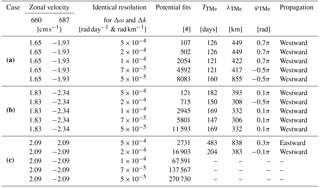Appendix B: Profiles of float 656 during the O2 low-oxygen event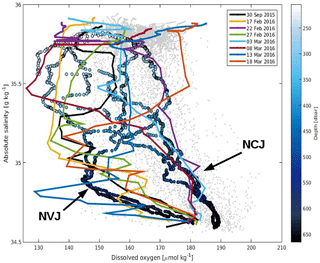Figure B1DOXY versus absolute salinity profiles of float 656 during O2 (colored lines) compared to characteristic NCJ and NVJ profiles made during the BIFURCATION cruise (scaled dots). The gray dots are the other measurements made by float 656. All the profiles are shown from the 1026 kg m−3 isopycnal to the 1030 kg m−3 one (i.e., between 200 and 650 m depth approximately).

Appendix C: Argo float profiles versus HYCOM re-analysis profiles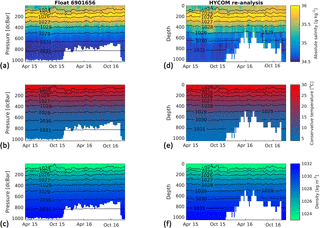Figure C1Profiles of (left side) float 656 and (right side) corresponding data from the HYCOM re-analysis over depth and time for (a, e) absolute salinity, (b, f) conservative temperature and (c, g) density. Every colored point corresponds to a measurement. The black lines indicate the isopycnals from 1024 to 1031 kg m−3.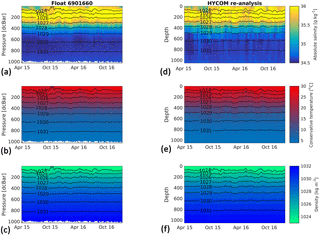Figure C2Profiles of (left side) float 660 and (right side) corresponding data from the HYCOM re-analysis over depth and time for (a, e) absolute salinity, (b, f) conservative temperature and (c, g) density. Every colored point corresponds to a measurement. The black lines indicate the isopycnals from 1024 to 1031 kg m−3.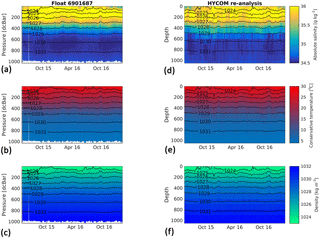Figure C3Profiles of (left side) float 687 and (right side) corresponding data from the HYCOM re-analysis over depth and time for (a, e) absolute salinity, (b, f) conservative temperature and (c, g) density. Every colored point corresponds to a measurement. The black lines indicate the isopycnals from 1024 to 1031 kg m−3.

Appendix D: Linear Rossby wave dispersion equation

We describe the Rossby wave in different cases: first, in a barotropic ocean, so the dispersion equation is

$\begin{array}{}\text{(D1)}& \mathit{\omega }=-\mathit{\beta }\frac{k}{{k}_{\mathrm{H}}^{\mathrm{2}}},\end{array}$

where ω is the frequency, k the wave number, kH the horizontal wave number of the wave and β the Rossby parameter. We place ourselves at 19 S, so $\mathit{\beta }=\mathrm{2.1}×{\mathrm{10}}^{-\mathrm{11}}$${\mathrm{m}}^{-\mathrm{1}}\phantom{\rule{0.125em}{0ex}}{\mathrm{s}}^{-\mathrm{1}}$. In our study we are not able to estimate the value of the meridional wave number; hence we neglect it and consider that kH=k (, also use this hypothesis in their study for calculation purposes). The equation becomes

$\begin{array}{}\text{(D2)}& \mathit{\omega }=\frac{\mathit{\beta }}{k}.\end{array}$

Second, we take a baroclinic ocean with two layers separated by the thermocline. Still considering kH=k, the dispersion equation is

$\begin{array}{}\text{(D3)}& \mathit{\omega }=\frac{-\mathit{\beta }k}{{k}^{\mathrm{2}}+\frac{{f}_{\mathrm{0}}^{\mathrm{2}}}{{c}^{\mathrm{2}}}},\end{array}$

where c is the phase speed and f0 the Coriolis parameter at 19 S, equal to $-\mathrm{4.7}×{\mathrm{10}}^{-\mathrm{5}}$s−1. In this case the phase speed of the wave is expressed by the following equation:

$\begin{array}{}\text{(D4)}& {c}^{\mathrm{2}}=\frac{g\mathrm{\Delta }\mathit{\rho }{h}_{\mathrm{1}}{h}_{\mathrm{2}}}{{\mathit{\rho }}_{\mathrm{2}}{h}_{\mathrm{1}}+{\mathit{\rho }}_{\mathrm{1}}{h}_{\mathrm{2}}},\end{array}$

where ρ1 and ρ2 are respectively the densities of the upper and lower ocean layer, $\mathrm{\Delta }\mathit{\rho }={\mathit{\rho }}_{\mathrm{2}}-{\mathit{\rho }}_{\mathrm{1}}$, and h1 and h2 the corresponding height of the layers. Using the climatology of the ISAS13 atlas and the HYCOM re-analysis, we make the calculations for four different cases (Table D1).

Table D1Characteristics of the layers for the baroclinic cases of the Rossby wave calculation.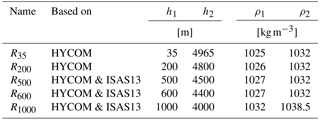Author contributions

SB led the main computations of the study as part of his master's internship at the University of Aix-Marseille under the supervision of AP and CM. All co-authors performed analyses and wrote the manuscript.

Competing interests

The authors declare that they have no conflict of interest.

Special issue statement

This article is part of the special issue “Interactions between planktonic organisms and biogeochemical cycles across trophic and N2 fixation gradients in the western tropical South Pacific Ocean: a multidisciplinary approach (OUTPACE experiment)”. It is not affiliated with a conference.

Acknowledgements

Special thanks to the officers and crew of the R/V L'Atalante who operated the OUTPACE cruise (https://doi.org/10.17600/15000900). Thanks to Luiz Neto (project: CR2017Z2MERO0; AMICO biogeochemistry; PI: Christel Pinazo) for developing a float profile toolbox and Alain Fumenia for the oxygen calibration of the OUTPACE floats. The authors are grateful for the support of the OUTPACE project (PIs: Thierry Moutin and Sophie Bonnet) funded by the French National Research Agency (ANR-14-CE01-0007-01), the LEFE-CyBER program (CNRS-INSU), the GOPS program (IRD), the European FEDER Fund under project 1166-39417 and TOSCA/CNES (PI: Andrea Doglioli, BC T23 ZBC 4500048836). We also thank ERC Remocean, the LEFE and MOM programs, and the Contrat de Projets Etat – Polynésie française for their financial supports to the THOT project led by Elodie Martinez (IRD). Simon Barbot thanks the physics team of MIO and the OUTPACE project for the funding of his internship, as well as the LATEX project (PIs: Anne Petrenko and Frédéric Diaz) for complementary support. Finally, the authors thank Xavier Carton for his proofreading and comments as well as Kelvin Richards and the two anonymous referees for their constructive comments.

Edited by: Kelvin Richards
Reviewed by: two anonymous referees

References

Argo: Argo float data and metadata from Global Data Assembly Centre (Argo GDAC), SEANOE, https://doi.org/10.17882/42182, 2000.

Belmadani, A., Concha, E., Donoso, D., Chaigneau, A., Colas, F., Maximenko, N., and Di Lorenzo, E.: Striations and preferred eddy tracks triggered by topographic steering of the background flow in the eastern South Pacific, J. Geophys. Res.-Oceans, 122, 2847–2870, 2017. a

Benavides, M., Berthelot, H., Duhamel, S., Raimbault, P., and Bonnet, S.: Dissolved organic matter uptake by Trichodesmium in the Southwest Pacific, Sci. Rep.-UK, 7, 41315, https://doi.org/10.1038/srep41315, 2017. a

Bishop, J. K. B. and Wood, T. J.: Year-round observations of carbon biomass and flux variability in the Southern Ocean, Global Biogeochem. Cy., 23, gB2019, https://doi.org/10.1029/2008GB003206, 2009. a

Bonnet, S., Caffin, M., Berthelot, H., and Moutin, T.: Hot spot of N2 fixation in the western tropical South Pacific pleads for a spatial decoupling between N2 fixation and denitrification, P. Natl. Acad. Sci. USA, 114, E2800–E2801, 2017. a

Boss, E., Swift, D., Taylor, L., Brickley, P., Zaneveld, R., Riser, S., Perry, M., and Strutton, P.: Observations of pigment and particle distributions in the western North Atlantic from an autonomous float and ocean color satellite, Limnol. Oceanogr., 53, 2112–2122, 2008. a

Bostock, H. C., Sutton, P. J., Williams, M. J., and Opdyke, B. N.: Reviewing the circulation and mixing of Antarctic Intermediate Water in the South Pacific using evidence from geochemical tracers and Argo float trajectories, Deep-Sea Res. Pt. I, 73, 84–98, 2013. a

Bouruet-Aubertot, P., Cuypers, Y., Doglioli, A., Caffin, M., Yohia, C., de Verneil, A., Petrenko, A., Lefèvre, D., Le Goff, H., Rougier, G., Picheral, M., and Moutin, T.: Longitudinal contrast in Turbulence along a  19 S section in the Pacific and its consequences on biogeochemical fluxes, Biogeosciences Discuss., https://doi.org/10.5194/bg-2018-170, in review, 2018. a

Brion, E. and Gaillard, F.: ISAS-Tool Version 6: User's manual, LPO-12-01, available at: http://archimer.ifremer.fr/doc/00115/22582/ (last access: 28 June 2018), 2012. a

Caffin, M., Moutin, T., Foster, R. A., Bouruet-Aubertot, P., Doglioli, A. M., Berthelot, H., Guieu, C., Grosso, O., Helias-Nunige, S., Leblond, N., Gimenez, A., Petrenko, A. A., de Verneil, A., and Bonnet, S.: N2 fixation as a dominant new N source in the western tropical South Pacific Ocean (OUTPACE cruise), Biogeosciences, 15, 2565–2585, https://doi.org/10.5194/bg-15-2565-2018, 2018. a

Cravatte, S., Kessler, W. S., and Marin, F.: Intermediate Zonal Jets in the Tropical Pacific Ocean Observed by Argo Floats, J. Phys. Oceanogr., 42, 1475–1485, 2012. a, b

Cravatte, S., Kestenare, E., Marin, F., Dutrieux, P., and Firing, E.: Subthermocline and Intermediate Zonal Currents in the Tropical Pacific Ocean: Paths and Vertical Structure, J. Phys. Oceanogr., 47, 2305–2324, https://doi.org/10.1175/JPO-D-17-0043.1, 2017. a

Davis, R. E.: Intermediate-depth circulation of the Indian and South Pacific Oceans measured by autonomous floats, J. Phys. Oceanogr., 35, 683–707, 2005. a

de Verneil, A., Rousselet, L., Doglioli, A. M., Petrenko, A. A., and Moutin, T.: The fate of a southwest Pacific bloom: gauging the impact of submesoscale vs. mesoscale circulation on biological gradients in the subtropics, Biogeosciences, 14, 3471–3486, https://doi.org/10.5194/bg-14-3471-2017, 2017. a

de Verneil, A., Rousselet, L., Doglioli, A. M., Petrenko, A. A., Maes, C., Bouruet-Aubertot, P., and Moutin, T.: OUTPACE long duration stations: physical variability, context of biogeochemical sampling, and evaluation of sampling strategy, Biogeosciences, 15, 2125–2147, https://doi.org/10.5194/bg-15-2125-2018, 2018. a

Flierl, G. R.: Particle motions in large-amplitude wave fields, Geophys. Astro. Fluid, 18, 39–74, 1981. a, b, c

Fumenia, A., Moutin, T., Bonnet, S., Benavides, M., Petrenko, A., Helias Nunige, S., and Maes, C.: Excess nitrogen as a marker of intense dinitrogen fixation in the Western Tropical South Pacific Ocean: impact on the thermocline waters of the South Pacific, Biogeosciences Discuss., https://doi.org/10.5194/bg-2017-557, in review, 2018. a, b, c

Gaillard, F., Reynaud, T., Thierry, V., Kolodziejczyk, N., and Von Schuckmann, K.: In situ–based reanalysis of the global ocean temperature and salinity with ISAS: Variability of the heat content and steric height, J. Climate, 29, 1305–1323, https://doi.org/10.1175/JCLI-D-15-0028.1, 2016. a

Ganachaud, A. and Wunsch, C.: Oceanic nutrient and oxygen transports and bounds on export production during the World Ocean Circulation Experiment, Global Biogeochem. Cy., 16, 1057, https://doi.org/10.1029/2000GB001333, 2002. a

Gasparin, F., Ganachaud, A., and Maes, C.: A western boundary current east of New Caledonia: Observed characteristics, Deep-Sea Res. Pt. I, 58, 956–969, 2011. a, b

Gasparin, F., Maes, C., Sudre, J., Garcon, V., and Ganachaud, A.: Water mass analysis of the Coral Sea through an Optimum Multiparameter method, J. Geophys. Res.-Oceans, 119, 7229–7244, 2014. a, b

IOCCG: Remote Sensing in Fisheries and Aquaculture, edited by: Forget, M.-H., Stuart, V., and Platt, T., Reports of the International Ocean-Colour Coordinating Group, No. 8, IOCCG, Dartmouth, Canada, 92–95, 2009. a

Las Heras, M. M. and Schlitzer, R.: On the importance of intermediate water flows for the global ocean overturning, J. Geophys. Res.-Oceans, 104, 15515–15536, 1999. a

Maes, C.: BIFURCATION cruise, RV Alis, https://doi.org/10.17600/12100100, 2012. a

Maharaj, A. M., Cipollini, P., Holbrook, N. J., Killworth, P. D., and Blundell, J. R.: An evaluation of the classical and extended Rossby wave theories in explaining spectral estimates of the first few baroclinic modes in the South Pacific Ocean, Ocean Dynam., 57, 173–187, 2007. a, b

Martinez, E., Claustre, H., Rodier, M., Poteau, A., Maes, C., Mignot, A., Taquet, M., Ponsonnet, C., and Maamaatuaiahutapu, K.: THOT (TaHitian Ocean Time series): a new kind of long-lasting deep-sea oceanographic station in the central South Pacific, 2015, in: Our common future under climate change, International scientific conference, Abstract book, 7–10 July 2015, Paris, France, 858 p., 2015. a, b, c

Maximenko, N. A., Bang, B., and Sasaki, H.: Observational evidence of alternating zonal jets in the world ocean, Geophys. Res. Lett., 32, L12607, https://doi.org/10.1029/2005GL022728, 2005.  a

Maximenko, N. A., Melnichenko, O. V., Niiler, P. P., and Sasaki, H.: Stationary mesoscale jet-like features in the ocean, Geophys. Res. Lett., 35, L08603, https://doi.org/10.1029/2008GL033267, 2008. a

Mendoza, C. and Mancho, A. M.: Hidden geometry of ocean flows, Phys. Rev. Lett., 105, 038501, https://doi.org/10.1103/PhysRevLett.105.038501, 2010. a

Mitchell, B.: Resolving spring bloom dynamics in the Sea of Japan, in: ALPS: Autonomous and Lagrangian Platforms and Sensors, Workshop Report, 26–27, available at: https://geo-prose.com/pdfs/alps_report.pdf (last access: 8 Febuary 2018), 2003. a

Moutin, T., Doglioli, A. M., de Verneil, A., and Bonnet, S.: Preface: The Oligotrophy to the UlTra-oligotrophy PACific Experiment (OUTPACE cruise, 18 February to 3 April 2015), Biogeosciences, 14, 3207–3220, https://doi.org/10.5194/bg-14-3207-2017, 2017. a, b, c

Ollitrault, M. and Colin de Verdière, A.: The ocean general circulation near 1000-m depth, J. Phys. Oceanogr., 44, 384–409, 2014. a, b, c

Ollitrault, M. and Rannou, J.-P.: ANDRO: An Argo-based deep displacement dataset, J. Atmos. Ocean. Tech., 30, 759–788, 2013. a, b, c

Phillips, H. and Bindoff, N.: On the nonequivalent barotropic structure of the Antarctic Circumpolar Current: An observational perspective, J. Geophys. Res.-Oceans, 119, 5221–5243, 2014. a

Rousselet, L., Doglioli, A., Maes, C., Blanke, B., and Petrenko, A.: Impacts of mesoscale activity on the water masses and circulation in the Coral Sea, J. Geophys. Res.-Oceans, 121, 7277–7289, 2016. a, b, c, d, e

Rousselet, L., de Verneil, A., Doglioli, A. M., Petrenko, A. A., Duhamel, S., Maes, C., and Blanke, B.: Large- to submesoscale surface circulation and its implications on biogeochemical/biological horizontal distributions during the OUTPACE cruise (southwest Pacific), Biogeosciences, 15, 2411–2431, https://doi.org/10.5194/bg-15-2411-2018, 2018. a, b

Sevellec, F., Colin de Verdiére, A., and Ollitrault, M.: Evolution of Intermediate Water Masses Based on Argo Float Displacements, J. Phys. Oceanogr., 47, 1569–1586, 2017. a

Webb, D. J.: Evidence for shallow zonal jets in the South Equatorial Current region of the southwest Pacific, J. Phys. Oceanogr., 30, 706–720, 2000. a